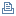Lotus Sandbox (1999-2007) <- domquery.info -> FeedBack & Questions@Formula validator / evaluator

Sandbox sample submitted by Ragnar Schierholz.

A toolbar icon that validates formula syntax and evaluates formulasCategory:Tools
Audience:Application Developer
Product:Notes/Domino
Release:6.0 (5.0 with slight modifications)
Platform:All Supported Platforms
Date Posted:02/20/2003
File Name:FormualValidator.txt
File Size:492 bytesClick here to download

Detailed Description:
This formula asks for a custom @formula as input and checks the syntax. If the formula is syntactically correct, it is being executed and the result is displayed. If not, the error message along with the column and the erroneous code snippet is displayed.

Since the original formula uses the new @CheckFormulaSyntax formula introduced with Notes 6, it won't run under R5. A stripped version is available for R5, with reduced functionality (i.e. no syntax check, thus clumsy error handling).

Code:
formula := @Prompt ( [OkCancelEdit]; "Evaluate"; "Enter a formula"; "" );
check := @CheckFormulaSyntax ( formula );
@If ( @Elements ( check ) = 1;
@Do (
result := @Eval ( formula );
@If ( @Elements ( result ) = 1;
@Prompt ( [Ok]; "Result"; @Text ( result ) );
@Prompt ( [Ok]; "Result"; @Implode ( result; " : " ) )
)
);
@Prompt ( [Ok]; "Errors in formula"; check + " (" + check + "): " +
check )
)

Code for R5 (not tested):
formula := @Prompt ( [OkCancelEdit]; "Evaluate"; "Enter a formula"; "" );
result := @Eval ( formula );
@If ( @Elements ( result ) = 1;
@Prompt ( [Ok]; "Result"; @Text ( result ) );
@Prompt ( [Ok]; "Result"; @Implode ( result; " : " ) )
)Document optionsPrint this pagePrint viewSearch

Sandbox viewsBy Application NameBy CategoryBy ProductBy AudienceBy DateBy SubmitterConferences

ResourcesdeveloperWorks: Lotus DownloadsalphaWorks Downloads